# 3. For each of the following production functions, graph a typical isoquant and determine whether the marginal rate of...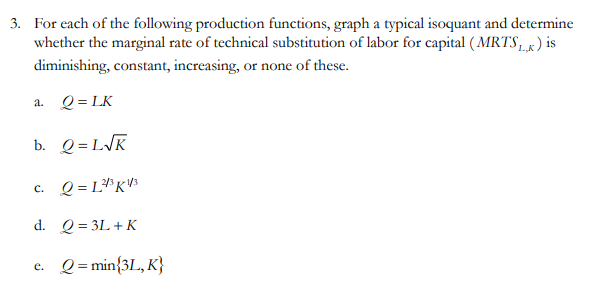Show transcribed image text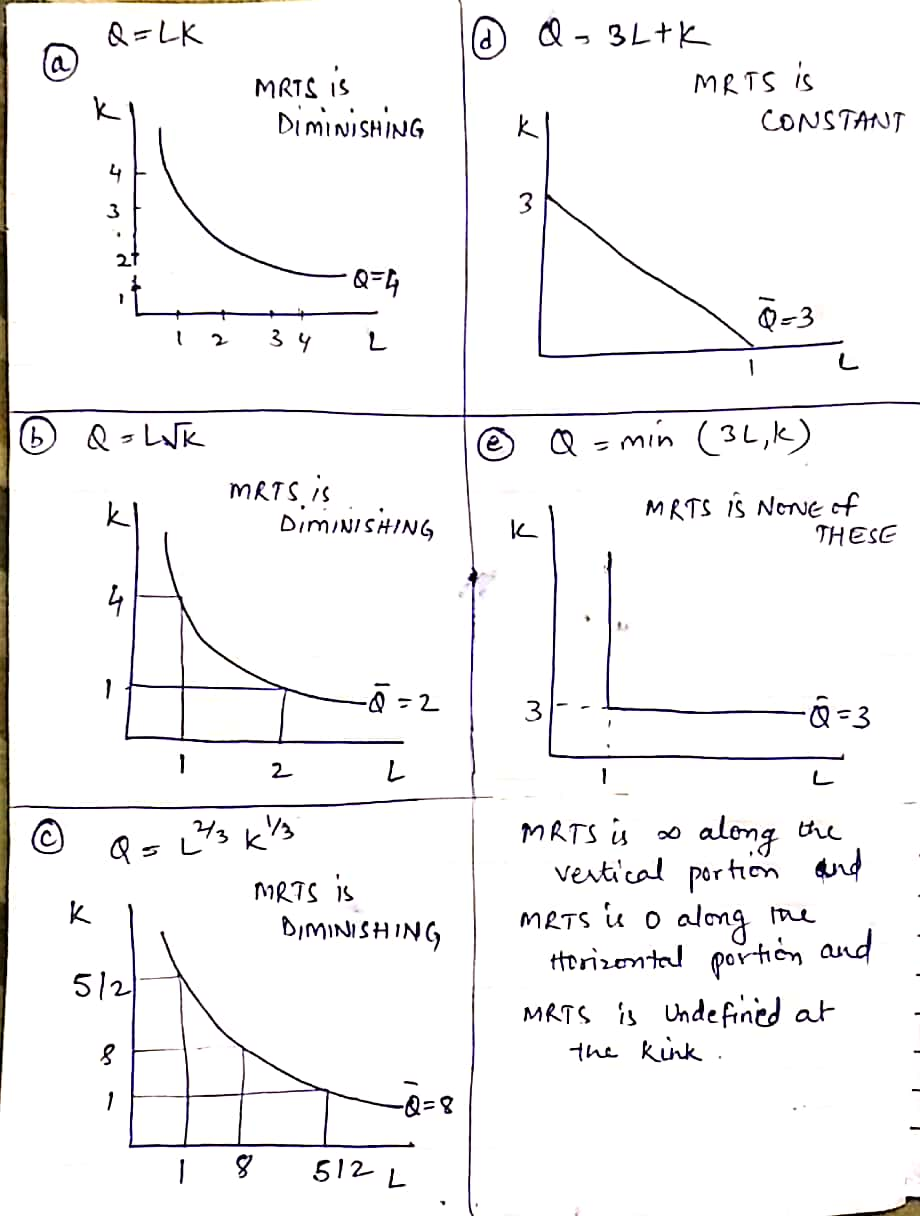#### Earn Coin

Coins can be redeemed for fabulous gifts.

Similar Homework Help Questions
• ### 1. A frequent assumption that economists make about production functions is that the marginal rate of...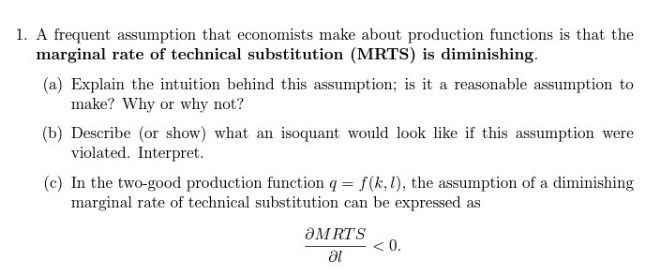1. A frequent assumption that economists make about production functions is that the marginal rate of technical substitution (MRTS) is diminishing. (a) Explain the intuition behind this assumption; is it a reasonable assumption to make? Why or why not? (b) Describe (or show) what an isoquant would look like if this assumption were violated. Interpret. (c) In the two-good production function q = f(k. 1), the assumption of a diminishing marginal rate of technical substitution can be expressed as SMRTS...

• ### For each of the following production functions, solve for the marginal products of each input and marginal rate of substitution.

For each of the following production functions, solve for the marginal products of each input and marginal rate of substitution. Then answer the following for each: does this production function exhibit diminishing marginal product of labour? Does this production function exhibit diminishing marginal product of capital? Does this production function exhibit constant, decreasing, or increasing returns to scale? Show all your work.(a) \(Q=L+K\)(b) \(Q=2 L^{2}+K^{2}\)(c) \(Q=L^{1 / 2} K^{1 / 2}\)

• ### 4. Consider the production functions given below: a. Suppose that the production function faced by a...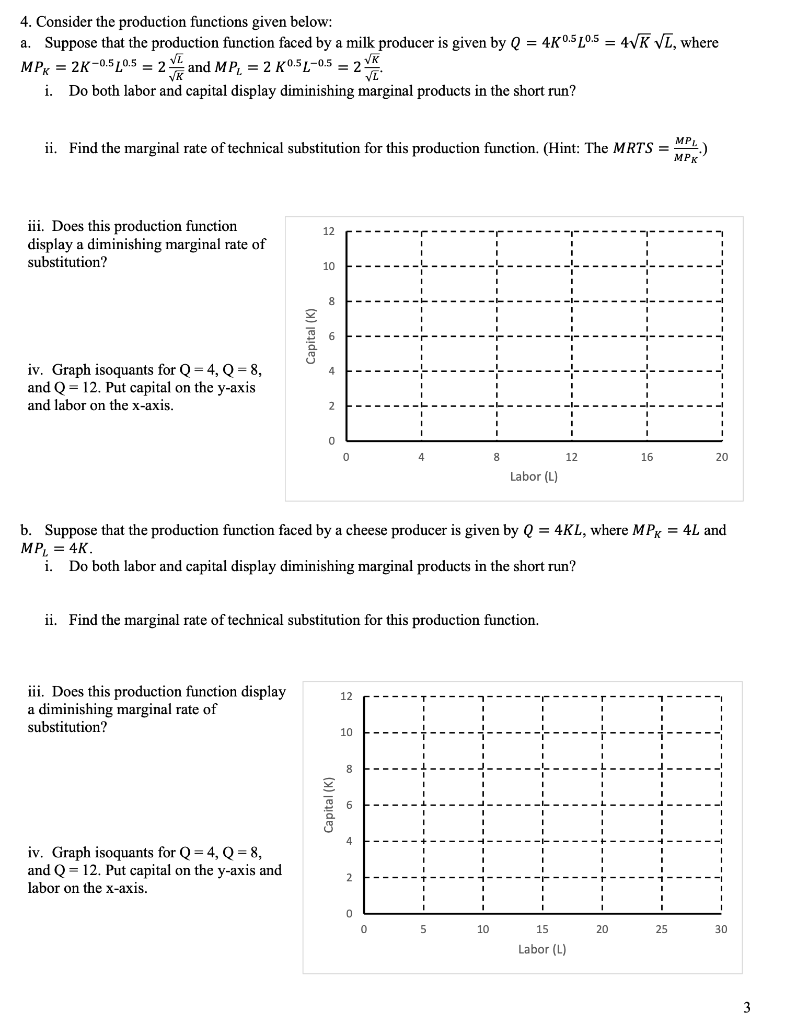4. Consider the production functions given below: a. Suppose that the production function faced by a milk producer is given by Q = 40.5 20.5 = 4VK VL, where MPx = 2K-0.5 20.5 = 2 and MP, = 2 K0.5L-05 = 2 * i. Do both labor and capital display diminishing marginal products in the short run? ii. Find the marginal rate of technical substitution for this production function. (Hint: The MRTS = 1) iii. Does this production function display...

• ### 4. Below are production functions that turn capital (K) and labor (L) into output. For each...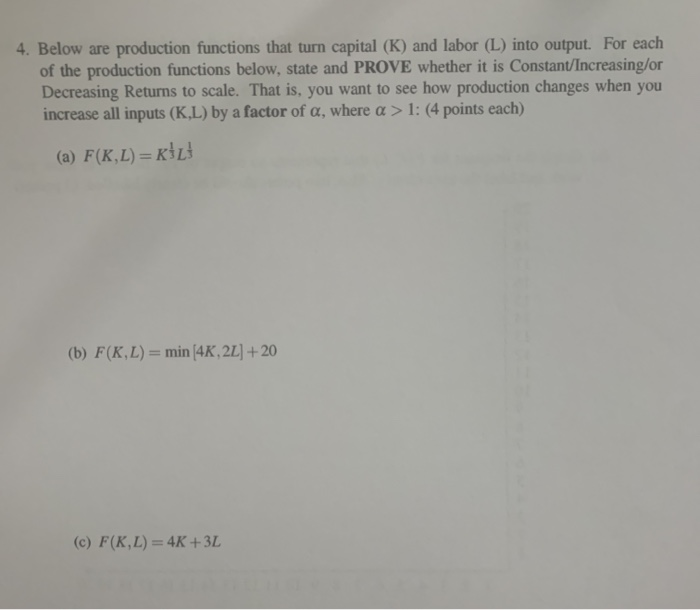4. Below are production functions that turn capital (K) and labor (L) into output. For each of the production functions below, state and PROVE whether it is Constant/Increasing/or Decreasing Returns to scale. That is, you want to see how production changes when you increase all inputs (K.L) by a factor of a, where a > 1: (4 points each) (a) F(K,L) =KİL (b) F(K,L) = min 4K, 2L] + 20 (c) F(K,L) = 4K +3L 5. For this problem you...

• ### 2. For each of the following production functions: Q = 3L2 +6K4 Q= V25KL Q =...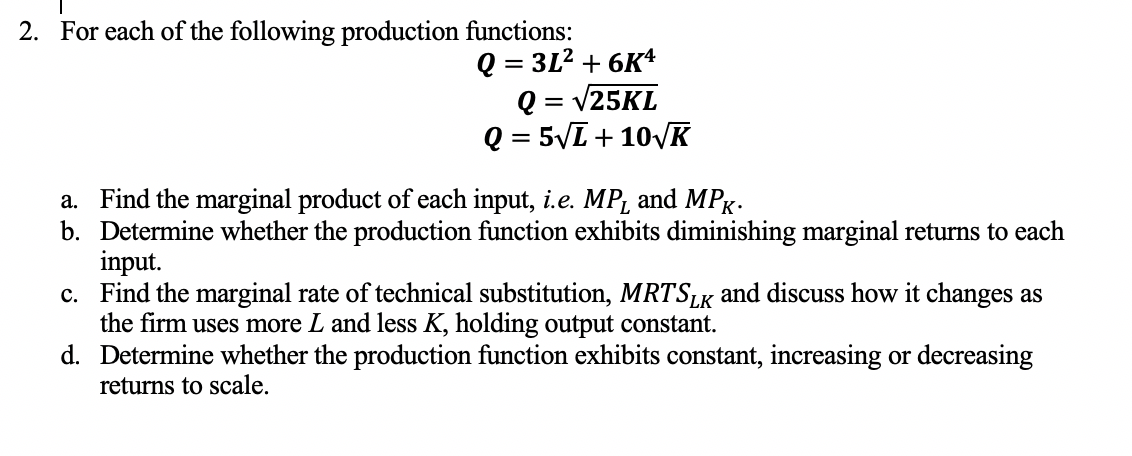2. For each of the following production functions: Q = 3L2 +6K4 Q= V25KL Q = 5V1 + 101K a. Find the marginal product of each input, i.e. MP, and MPx: b. Determine whether the production function exhibits diminishing marginal returns to each input. c. Find the marginal rate of technical substitution, MRTSLK and discuss how it changes as the firm uses more L and less K, holding output constant. d. Determine whether the production function exhibits constant, increasing or...

• ### Consider the following Cobb-Douglas production function, where α Find the equation for the isoquant when Q...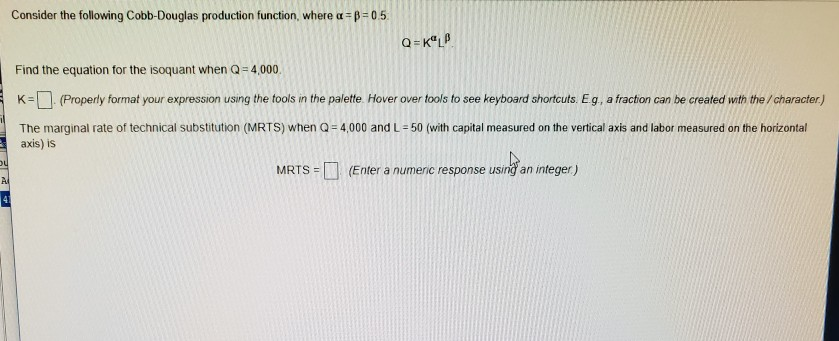Consider the following Cobb-Douglas production function, where α Find the equation for the isoquant when Q 4,000. K-(Properly format your expression using the fools in the paiette. Hover over tools to see keyboard shortcuts Eg, a fraction can be created with the /character) The marginal rate of technical substitution (MRTS) when Q 4,000 and L-50 (with capital measured on the vertical axis and labor measured on the horizontal axis) is MRTS (Enter a numenic response using an integer)

• ### Which of the following are characteristics of a typical isoquant

Which of the following are characteristics of a typical isoquant? a. All input combinations on the isoquant will produce the same level of output. b. The marginal rate of technical substitution decreases as more labor is substituted forless capital. c. a change in input prices shifts the isoquant map. d. both a and b e. none of the above.

• ### Consider the following Cobb Douglas production function, where =0.5 Q=KLP Find the equation for the isoquant...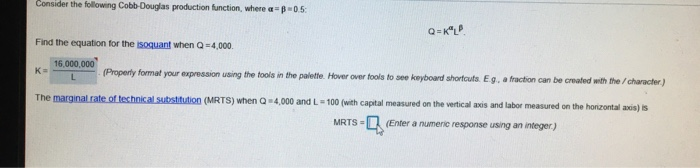Consider the following Cobb Douglas production function, where =0.5 Q=KLP Find the equation for the isoquant when Q=4,000 16.000.000 (Property format your expression using the tools in the palette. Hover over fools to see keyboard shortcuts. Eg, a fraction can be created with the character.) The marginal rate of technical substitution (MRTS) when Q ) 4,000 and L - 100 (with capital measured on the vertical axis and labor measured on the horizontal 2 MRTS - (Enter a numeric response...

• ### 3. Let q = Lākā denote the production function for a firm making long-run decisions, that...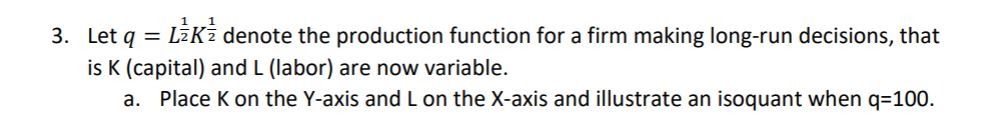3. Let q = Lākā denote the production function for a firm making long-run decisions, that is K (capital) and L (labor) are now variable. a. Place k on the Y-axis and L on the X-axis and illustrate an isoquant when q=100. b. Derive an expression for the MRTS (the marginal rate of technical substitution) for any level of q.

• ### Given production function Q-fCK, L)-8KL +、/L where K is capital and L is labor. b. Define...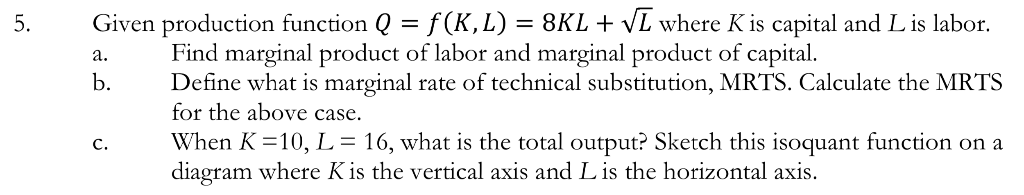Given production function Q-fCK, L)-8KL +、/L where K is capital and L is labor. b. Define what is marginal rate of technical substitution, MRTS. Calculate the MRTS c. When K = 10, L-16, what is the total output? Sketch this isoquant function on a Find marginal product of labor and marginal product of capital. for the above case. diagram where Kis the vertical axis and L is the horizontal axis

Free Homework App